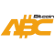Bitcoin ABC  0.22.13 P2P Digital Currency
grasberg Namespace Reference

## Functions

int64_t computeTargetBlockTime (const CBlockIndex *pindexPrev, const CChainParams &chainParams)
Compute the block time we are aiming for. More...

uint32_t deterministicExp2 (const uint32_t n)
Computes exp2(n) = 2^32 * (2^(n/2^32) - 1) More...

## ◆ computeTargetBlockTime()

 int64_t grasberg::computeTargetBlockTime ( const CBlockIndex * pindexPrev, const CChainParams & chainParams )

Compute the block time we are aiming for.

The split between the integer and decimal part of x32 does not happen like one might intuitively think using "regular math". This is because "computer math" differs in ways that might be subtle, but nevertheless are very important. The integer part is ALWAYS rounded down. For instance, 0.3 is split as 0 + 0.3, which is intuitive. But -0.3 is split as -1 + 0.7 , which means we need to multiply by 2^-1 when x32 is negative. This is implemented as a right shift.

Definition at line 108 of file grasberg.cpp.

Here is the call graph for this function:
Here is the caller graph for this function:

## ◆ deterministicExp2()

 uint32_t grasberg::deterministicExp2 ( const uint32_t n )

Computes exp2(n) = 2^32 * (2^(n/2^32) - 1)

/!\ This function is a consensus dependent aproximation. The error due to the aproximation is at most 0.0000000075 , which is enough for a wide variety of applications. It is important to not modify the behavior of this function, for instance in the hopes of making it more precise, as it woud break consensus and might cause a split.

Rescale the computation depending on n for better precision. We use the MSB to form 4 buckets.

Rescale on the range [0 .. 0.25[ via: exp2(n) = 2^32 * 2^(n/2^32) = 2^32 * 2^((n - d)/2^32 + d/2^32) = 2^32 * 2^(d/2^32) * 2^((n - d)/2^32) Using x = n - d: exp2(n) = 2^32 * 2^(d/2^32) * 2^(x/2^32)

Definition at line 140 of file grasberg.cpp.

Here is the caller graph for this function: